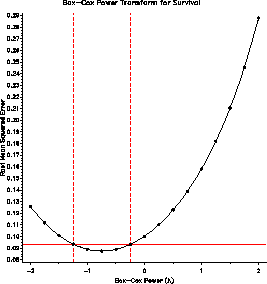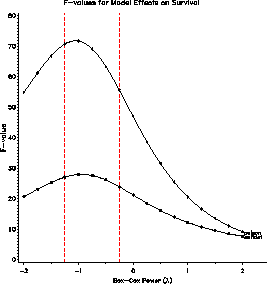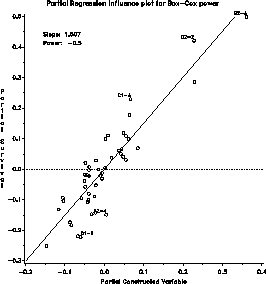boxglm Power transformations by Box-Cox method for GLMs boxglm

# SAS Macro Programs: boxglm

\$Version: 1.1 (10 Dec 1991)
Michael Friendly
York University

## The boxglm macro (get boxglm.sas)

### Power transformations by Box-Cox method for GLMs

The boxglm macro finds power transformations of the response variable in a general linear model by the Box-Cox method, with graphic display of the maximum likelihood solution (RMSE plot), F-values for model effects (EFFECT plot), and the influence of observations on choice of power (INFL plot). The program produces printer plots by default, and can optionally produce high-resolution versions of any of these plots.

### Method

The program uses transforms the response to all powers from the LOPOWER= value to the HIPOWER= value, and fits a linear model using PROC GLM for each, extracting values to an output dataset from which the plots are drawn.

The influence plot also implements a score test for the power transformation due to Atkinson, which provides an alternative estimate of the power transformation. based on power = 1 - slope of the fitted line in the partial regression plot for a constructed variable.

## Usage

boxglm is a macro program. Values must be supplied for the RESP= and MODEL= parameters.

The arguments may be listed within parentheses in any order, separated by commas. For example:

```   %boxglm(resp=responsevariable, model=predictors, ..., )
```

### Parameters

RESP=
The name of the response variable for analysis.
MODEL=
The independent variables in the model, i.e., the terms on the right side of the = sign in the MODEL statement for PROC GLM. The MODEL= argument should be spelled out completely, rather than using abbreviated 'bar' notation.
CLASS=
Specifies the MODEL= variables which are classification factors rather than continuous variables.
DATA=_LAST_
The name of the data set holding the response and predictor variables. (Default: most recently created)
ID=
The name of an ID variable for observations
OUT=_DATA_
The name of an output dataset to contain the transformed response. This dataset contains all original variables, with the transformed response replacing the original variable.
OUTPLOT=_PLOT_
The name of the output data set containing _RMSE_, and t-values for each effect in the model, with one observation for each power value tried. PPLOT=RMSE EFFECT INFL
Which printer plots should be produced? One or more of RMSE, EFFECT, and INFL, or NONE.
GPLOT=NONE
Which high-resolution (PROC GPLOT) plots should be produced? One or more of RMSE, EFFECT, and INFL, or NONE.
LOPOWER=-2
low value for power
HIPOWER=2
high value for power
NPOWER=21
number of power values in the interval LOPOWER to HIPOWER
CONF=.95
confidence coefficient for the confidence interval for the power.

### Example

The example finds power transformations for the SURVIVAL variable in a dataset relating survival time to type of poison and antidote used.
```data poisons;
input antidote \$ poison @;
label survival='Survival time';
length id \$4;
do rep = 1 to 4;
id = trim(antidote)||trim(put(poison,1.))||'-'||put(rep,1.);
input survival @;
output;
end;
cards;
A 1  .31  .45  .46  .43
A 2  .36  .29  .40  .23
A 3  .22  .21  .18  .23
B 1  .82 1.10  .88  .72
B 2  .92  .61  .49 1.24
B 3  .30  .37  .38  .29
C 1  .43  .45  .63  .76
C 2  .44  .35  .31  .40
C 3  .23  .25  .24  .22
D 1  .45  .71  .66  .62
D 2  .56 1.02  .71  .38
D 3  .30  .36  .31  .33
;
*include macros(boxglm);
%boxglm(data=poisons,
resp=Survival,
model=antidote poison,
class=antidote poison,
id=id,
gplot=RMSE EFFECT INFL,
npower=17, conf=.99);
```
The plot of RMSE vs. lambda (power) indicates power = -1 / sqrt(SURVIVAL) as the maximum likelihood estimate, but power = -1 / SURVIVAL == survival rate is within the confidence interval.

The EFFECT plot indicates that the significance of partial F-tests are unaffected by the choice of power. The influence plot indicates that a few observations have a large leverage, but none is influential in determining the choice of power.### See also

boxcox Power transformations by Box-Cox method
boxtid Power transformations by Box-Tidwell method
outlier Robust multivariate outlier detection
resline Resistant line for bivariate data
stars Diagnostic plots for transformations to symmetry